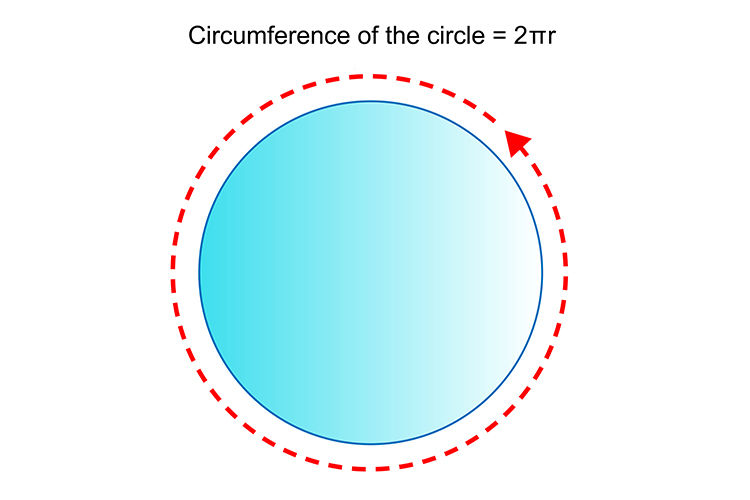# Radians and the good news

The good news is you don’t have to remember

1 radian =57.296^@

Because it is so easy to work out what the degrees are.So if:

360^@=100% of circle

2pir=360^@

1r=x^@

(Look at our section on percentages)

 (2pir)/r = 360/x x = (360timesr)/(2pir) x = (360times cancelr)/(2pi cancelr) x = 360/(2pi) x = 57.296^@